XOR problem theory

Let's imagine neurons that have attributes as follow:
- they are set in one layer
- each of them has its own polarity (by the polarity we mean b1 weight which leads from single value signal)
- each of them has its own weights Wij that lead from xj inputs
This structure of neurons with their attributes form a single-layer neural network. Above parameters are set in the learning process of a network (output yi signals are adjusting themselves to expected ui set signals) (Fig.1). This type of network has limited abilities. For example, there is a problem with XOR function implementation. (Assume that activation function is step function signal).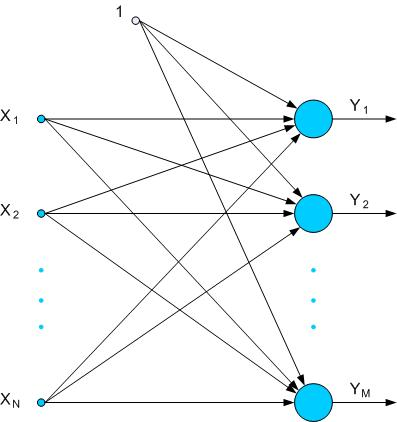Fig. 1. Single-layer network

The possibility of learning process of neural network is defined by linear separity of teaching data (one line separates set of data that represents u=1, and that represents u=0). These conditions are fulfilled by functions such as OR or AND.

For example, AND function has a following set of teaching vectors (Tab. 1.)

 x1 x2 u 0 0 0 0 1 0 1 0 0 1 1 1

Tab. 1. Set of teaching vectors of AND function

The neural network that implements such a function is made of one output neuron with two inputs x1, x2 and b1 polarity (Fig. 2).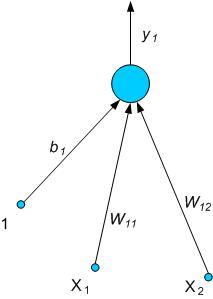Fig. 2. Neural network that can implement AND function

Assume that during teaching process y1 = f ( W11x1 + W12x2 + b1 ) = u1 which is ilustrated on Fig. 3.Fig. 3. Linear separity in case of AND function

As it's seen in Tab. 1, we should receive '1' as output signal only in (1,1) point. The equation of line that implements linear separity is u1 = W11x1 + W12x2 + b1. So we can match this line to obtain linear separity by finding suitable coefficients of the line (W11, W12 i b1). As we can see of Fig. 3., it's no problem for AND function.

Linear separity can be no longer used with XOR function (teaching vectors of this function are shown in Tab. 2.).

 x1 x2 u 0 0 0 0 1 1 1 0 1 1 1 0

Tab. 2. Set of teaching vectors of XOR function

It means that it's not possible to find a line which separates data space to space with output signal - 0, and space with output signal - 1 (Fig. 4). Inside the oval area signal on output is '1'. Outside of this area, output signal equals '0'. It's not possible to make it by one line.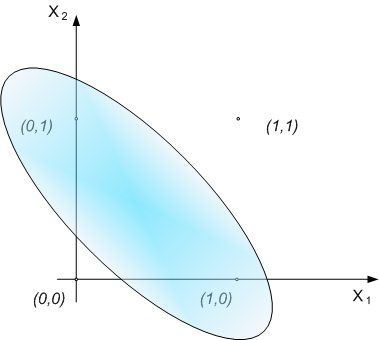Fig. 4. Data space of XOR function

The coefficients of this line and the weights W11, W12 and b1make no affect to impossibility of using linear separity. So we can't implement XOR function by one perceptron.

The solve of this problem is an extension of the network in the way that one added neuron in the layer creates new network. Neurons in this network have weights that implement division of space as below:

1) for 1st neuron

u1 = W11x1 + W12x 2 + b1 > 0
u1 = W21x1 + W22x 2 + b1 < 0

2) for 2nd neuron

u2 = W21x1 + W22x 2 + b2 > 0
u2 = W21x1 + W22x 2 + b2 < 0

The division should be like in Figure No 5.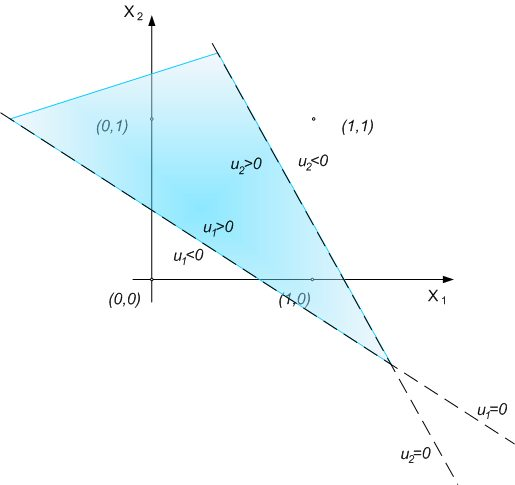Fig. 5. The way of implementation of XOR function by multilayer neural network

After adding the next layer with neuron, it's possible to make logical sum. On the Fig. 5 we can see it as a common area of sets u1>0 and u2>0.
Fig. 6 shows full multilayer neural network structure that can implement XOR function. Each additional neuron makes possible to create linear division on ui>0 and ui<0 border that depends on neuron weights. Output layer is the layer that is combination of smaller areas in which was divided input area (by additional neuron).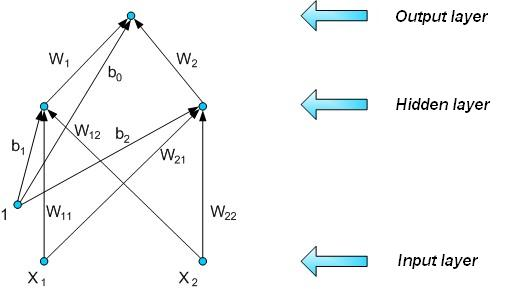Fig. 6. Structure of a network that has ability to implement XOR function